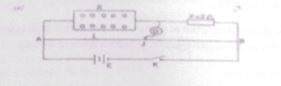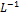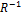Physics Paper 1B, May/June 2012
 Questions: 1 2 3 Main
Weakness/Remedies
Strength

Question 3bYou are provided with a meter bridge, standard resistor X galvanometer. jockey, cells, a key resistance box and some connecting wires.

1. Connect the circuit as shown above.
2. With R set to 10 Q obtain a balance point J on VB
3. Measure and record length AJ =L
4. Evaluateand5. Repeat the procedure with values of R = 20 Ω, 30 Ω,  40 Ω, 50 Ω and 6O Ω
6. In each case, measure and record L and evaluateand8.  Plot a graph withon the vertical axis andon the horizontal avis
9. Determine the slope, s, of the graph and the intercept, i. on the vertical axis
10. Evaluate the quantities.

11. (α) Q, = 100s,
(β) Q2       = i-1

12. State two precautions taken to ensure accurate results.

(b) (i) State Ohm's law

1. Calculate the resistivity of a wire of length 1 0m* diameter 7 0 x 10“* m and electrical resistance of 1.00

_____________________________________________________________________________________________________
observation

This was well attempted by many candidates and the performance was average Many candidates did not evaluate I1 and R 1 to the required 3 decimal places The determination of the intercept on the vertical axis, gave most candidates problem because they disregarded the instruction which says they should start the graph from the origin (0 0)
Part (b)(i) on Ohm s law was well stated but b(ii) which was a numerical part on resistivity was poorly handled